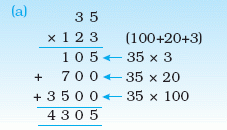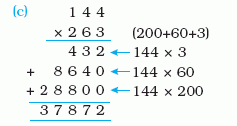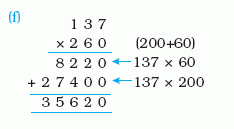Email us to get an instant 20% discount on highly effective K-12 Math & English kwizNET Programs!

Online Quiz (WorksheetABCD)

Questions Per Quiz = 2 4 6 8 10

MEAP Preparation - Grade 6 Mathematics2.62 Multiplication - Three Digits (WIZ Math)

 Multiplying 2 digit and 3 digit numbersMultiplying 3 digit and 2 digit numbersMultiplying 3 digit and 3 digit numbersDirections: Multiply the numbers shown in the question. Use a separate sheet to show your work and write the answer in the box.
 Question 1: 653 * 821

Question 2: This question is available to subscribers only!

Question 3: This question is available to subscribers only!

Question 4: This question is available to subscribers only!

Question 5: This question is available to subscribers only!

Question 6: This question is available to subscribers only!